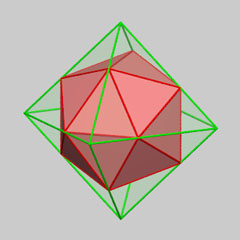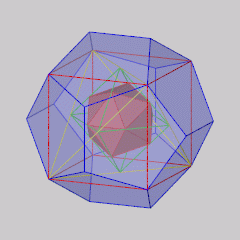A regular icosahedron inscribed in a regular octahedron

If one devides (see plate) the edges of a regular octahedron in an appropriate way using the "golden section", it can be shown that the twelve division points are the vertices of a regular icosahedron!An interesting combination of the five regular polyhedra

Eight of the vertices of a regular dodecahedron are the vertices of a cube. In this cube we inscribe a regular tetrahedron. The midpoints of the edges of this tetrahedron are the vertices of a regular octahedron. In this octahedron we construct a regular icosahedron using the method explained before. In this way we obtain a very nice combination of the five regular polyhedra.